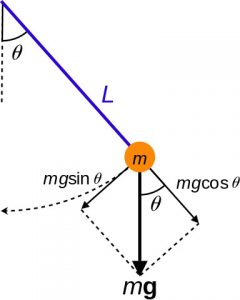# Types of mathematical models

In engineering, mathematical modeling of systems brings several advantages, in the design phase of any project, because:

• allows a better understanding of how the systems works
• gives the system’s response (output) for different input stimulus (e.g. step, ramp inputs)
• allows the user to understand the limitations of the system in extreme working conditions (e.g maximum speed and torque)
• the model can be used for closed-loop controller design (e.g. PID)

The mathematical model of a system is basically a set of equations which are describing the behavior of the system and the relationship between the system’s variables.

A particular mathematical model of a system can not be used for general applications but only for specialized applications. Building an universal mathematical model of a system, that can fit all purposes, it’s very difficult, uneconomical and impractical. For example, the same mathematical model of a vehicle, can not be used for studies of structural behavior, vibration analysis and fuel consumption optimization. For each area of interest, a particular mathematical model needs to be developed, in order to capture the relevant physical phenomena.

### Nonlinear models

A nonlinear mathematical model contains nonlinear differential equations. A nonlinear model is a model for which the principle of superposition does not apply.

An example of nonlinear model is the simple pendulum.Image: Generalized coordinates of a simple pendulum

The differential equation describing the motion of the pendulum is:

$\frac {d^{2}\theta }{dt^{2}} + \frac {g}{L} \sin \theta =0 \tag{1}$

where:
L [m] – length of the pendulum
g [m/s2] – gravitational acceleration

Notice that the dependent variable θ contains a nonlinear function, the trigonometric function sin(θ). That is why the differential equation is nonlinear.

### Linear models

A linear mathematical model is governed by linear differential equations. A linear model is a model for which the superposition principle can be applied.

The superposition principle states that, for a linear system, the response in time caused by two or more stimuli is the sum of the responses that would have been caused by each stimulus individually. This means that if input u1 produces response y1 and input u2 produces response y2 then input (u1 + u2) produces response (y1 + y2).

$y(u_1 + u_2) = y(u_1) + y(u_2)$

For the pendulum example above is we consider that the angle θ has small values, then:

$\sin \theta \approx \theta$

Replacing sin(θ) in equation (1), we get:

$\frac {d^2 \theta}{dt^2}+ \frac {g}{L} \theta =0$

which is a linear differential equation for a harmonic oscillator.

### Distributed models

In case of a distributed parameter system, its states depend on both time and location. Therefore a distributed system can be described by partial differential equations (PDE).

For example a homogeneous plate, under pure bending, is governed by the following partial differential equation:

$\frac{\partial^4 w}{\partial x_1^4} + 2 \frac{\partial^4 w}{\partial x_1^2 \partial x_2^2} + \frac{\partial^4 w}{\partial x_2^4} = 0$

Distribute models are used, for example, in vehicle structure analysis, using finite element analysis (FEA) method.

### Lumped models

In the case of lumped parameter systems, the states are concentrated in single points and are not spatially distributed. Therefore, lumped systems are described using ordinary differential equations (ODE).

An example of lumped parameter system is a spring mass damper system.

The differential equation describing the movement of the mass m is:

$m \cdot \frac{d^2x}{dt^2} + c \cdot \frac{dx}{dt} + k \cdot x = F(t)$

where:

m [kg] – wheel mass
c [N·s/m] – viscous damping coefficient
k [N/m] – spring stiffness coefficient
x [m] – wheel displacement (position)
dx/dt [m/s] – wheel vertical speed
d2x/dt2 [m/s2] – wheel vertical acceleration
F(t) [N] – input force

### Time-varying models

Time-varying models are described by differential equations with time-varying coefficients. For example, for the spring mass damper system above, if we consider that the viscous damping coefficient is not constant, but a function of time c(t), the differential equation becomes:

$m \cdot \frac{d^2x}{dt^2} + c(t) \cdot \frac{dx}{dt} + k \cdot x = F(t)$

### Stationary models

Stationary models have parameters which are constant in time. These models are governed by differential equations with constant coefficients.

Example of a stationary model is the RL circuit differential equation:

$L \cdot \frac{di}{dt} + R \cdot i = E$

where:

E [V] – battery voltage
R [Ω] – resistance
L [H] – inductance
i [A] – electric current

### Continuous models

Continuous models are described by differential equations, in which dependent variables (x) are defined over continuous range of independent variables (t). All of the above examples are continuous-time models.

Example: simple mass spring system, with the governing differential equation:

$m \frac{d^2x}{dt^2}+kx=0$

where:

m [kg] – mass
k [N/m] – spring stiffness (coefficient)

### Discrete models

Discrete models are described by time-difference equations, in which dependent variables (x) are defined only for distinct values (k) of independent variables (t).

The discrete form of the continuous spring mass system is:

$x[k+2]=2x[k+1]-C x[k]$

where C is a coefficient depending on the mass m and spring stiffness k.

For any questions, observations and queries regarding this article, use the comment form below.

Don’t forget to Like, Share and Subscribe!